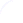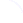Search
 Submission Procedure share: |available in:   PDF (166 kB) PS (58 kB)

get:
 Similar Docs BibTeX Write a comment
get:

DOI:   10.3217/jucs-002-08-0570

### The Edge-Flipping Distance of Triangulations

Sabine Hanke (Institut für Informatik, Universität Freiburg, Germany)

Thomas Ottmann (Institut für Informatik, Universität Freiburg, Germany)

Sven Schuierer (Institut für Informatik, Universität Freiburg, Germany)

Abstract: An edge-flipping operation in a triangulation T of a set of points in the plane is a local restructuring that changes T into a triangulation that differs from T in exactly one edge. The edge-flipping distance between two triangulations of the same set of points is the minimum number of edge-flipping operations needed to convert one into the other. In the context of computing the rotation distance of binary trees Sleator, Tarjan, and Thurston show an upper bound of 2n - 10 on the maximum edge-flipping distance between triangulations of convex polygons with n nodes, n > 12. Using volumetric arguments in hyperbolic 3-space they prove that the bound is tight. In this paper we establish an upper bound on the edge-flipping distance between triangulations of a general finite set of points in the plane by showing that no more edge-flipping operations than the number of intersections between the edges of two triangulations are needed to transform these triangulations into another, and we present an algorithm that computes such a sequence of edge-flipping operations.

Keywords: edge-flipping distance, edge-flipping operation, flip, rotation distance, triangulation

Categories: F.2.2, G.2.2